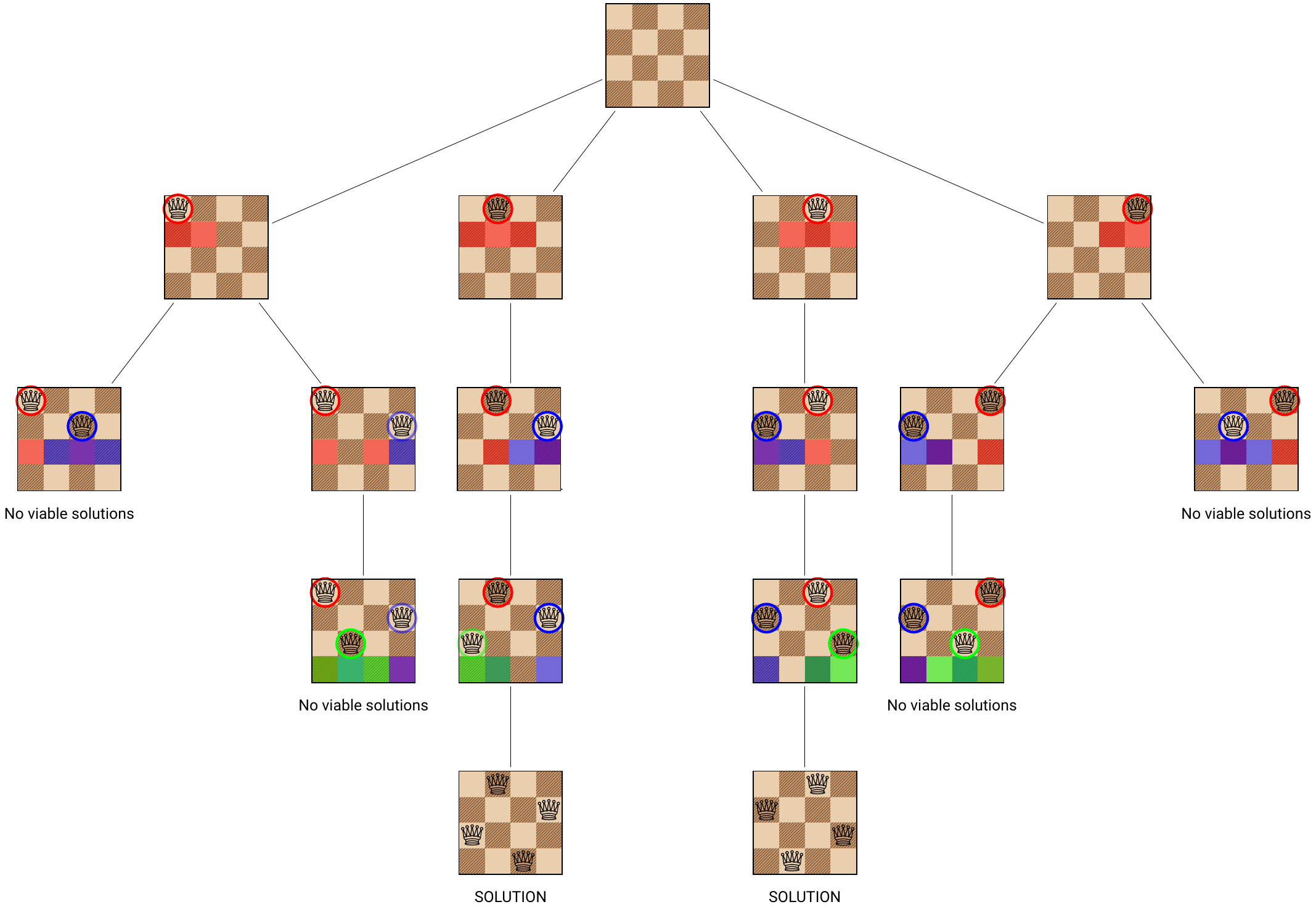# Backtracking Interview Essentials

Backtracking is a technique used to build up to a solution to a problem incrementally. These “partial solutions” can be phrased in terms of a sequence of decisions. Once it is determined that a “partial solution” is not viable (i.e. no subsequent decision can convert it into a solution) then the backtracking algorithm retraces its step to the last viable “partial solution” and tries again.

Visualizing the decisions as a tree, backtracking has many of the same properties of depth-first search. The difference is that depth-first search will guarantee that it visits every node until it finds a solution, whereas backtracking doesn’t visit branches that are not viable.

Because backtracking breaks problems into smaller subproblems, it is often combined with dynamic programming, or a divide-and-conquer approach.

Common interview tasks that use backtracking are crossword puzzles, Sudoku solvers, splitting strings, and cryptarithmetic puzzles.

#### Important Concepts

• State: A “partial solution” to the problem.
• Rejection criterion: A function that rejects a partial solution as “unrecoverable”. i. e. no sequence of subsequent decisions can turn this state into a solution. Without rejection criteria, backtracking is the same as depth-first search.
• Viable: A state that may still lead to a solution. This reflects what is known “at this stage”. All states that fail the rejection criteria are not viable. If we have a state that is initially viable, and find that all paths to leaves eventually terminate at a state that fail the rejection criteria, the state will become non-viable.
• Heuristic: Backtracking will (eventually) look at all the different viable nodes by recursively applying all the possible decisions. A heuristic is a quick way of ordering which decisions are likely to be the best ones at each stage, so that they get evaluated first. The goal is to find a solution early (if one exists).
• Pruning: The concept of determining that there are no nodes / states along a particular branch, so it is not worth visiting those nodes.

#### Related Concepts

Here are some concepts that will help you implement a backtracking algorithm.

• Depth-first search:
A backtracking algorithm is conceptually very similar to depth-first search (DFS). A DFS will “roll back” the current state to a node up the tree once it reaches a leaf node, and is guaranteed to find a solution (or search every node). Backtracking can be thought of as DFS with early pruning.
• Recursion:
Backtracking is often implemented using recursion, so it helps to be familiar with how to write recursive functions.
• Stacks:
If you need a lot of nested recursive calls, trying to use simple recursion might result in a stack overflow error. You can mimic recursion by using a stack data structure.

#### Generalized Algorithm

In the algorithms below, it is assumed that each decision is irreversible, so there is only one path to each state. If modeling a game like chess, you would have to be more careful, as it is possible to arrive at the same state multiple different ways. If it is possible to reach each state multiple ways, then we would also need to keep track of which states we had already visited.

A recursive implementation of a backtracking algorithm takes the general form

``````function doBacktrack( current ):
if current is a solution:
return current
for each decision d from current:
new_state <- state obtained from current by making decision d
if new_state is viable:
sol <- doBacktrack(new_state)
if sol is not None:
return sol
# indicate there is no solution
return None
``````

In some languages, there is a limit to how many nested recursive calls you are allowed to make. Exceeding this limit raises a stack overflow error. (Python is notorious for defaulting to a low number of nested recursive calls.) Behind the scenes, calling a function recursively pushes all your variables to the call stack. We can get around this limit by implementing our own stack.

``````function doBacktrackIterative(start):
stack <- initialize a stack
stack.push(start)

while (stack not empty):
current = stack.pop()

if current is a solution:
return current

for each decision d from current:
new_state <- state obtained from current by making decision d
if new_state is viable:
stack.push(new_state)

return None
``````

#### For An Interview, Expect To See Problems Like…

1. Placing n queens on an n x n chess board so no queen can be taken

We know that we need one queen in every row, and one queen in every column.

• Initial state: An empty board
• The decision: The decision at stage i is which column to put the queen on row i into.
• Rejection criterion: Reject all configurations that do not allow any queen on any of the lower rows. (Adding more queens to the board will not prevent this row from being blocked.)

Here are the states that the backtracking algorithm explores for a 4×4 board. Note that naively there are 4! = 24 complete configurations with 4 queens on the board, if we place the queens row-by-row, and avoid reusing a column that is already used. With backtracking, many configurations are eliminated early. This algorithm shows all solutions, instead of stopping at the first one found.1. Finding a subset of a set of positive integers S that sum to a particular number

We have a set of numbers S = {10, 7, 5, 18, 12, 20, 8}, and a target value T = 23. The goal is to find a subset of s of S whose elements sum to the target value, or report that such a sum doesn’t exist.

• Initial state: The subset s is empty
• The decision: Placing an element from S – s into s.
• Rejection criterion: If the sum of elements in s so far is greater than 18 (and is not a solution), then this solution is infeasible. This is because 5 is the smallest element in S.
1. Finding the best possible move in a game

A generic approach for finding the best strategy (sequence of moves) in a game is:

• Initial state: The position of the pieces at the beginning of the game.
• The decision: Making a valid move.
• Rejection criterion: Varies a lot by game.
Tagged on: